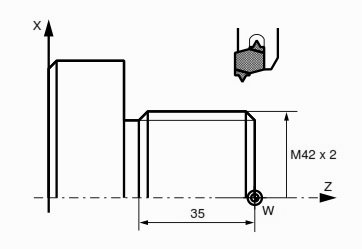Here is a CNC program example which shows how CNC machinists can cut external threads with Sinumerik L97 threading cycle.

## Sinumerik L97 Thread Cutting Cycle CNC Program ExampleSinumerik L97 Thread Cutting Cycle Program Example

```%97
N05 G95 G0 X50 Z10 D01 T01 S1000 M04
N10 R20=2 R21=42 R22=0 R23=0
R24=-1.23 R25=0 R26=10 R27=3
R28=5 R29=30 R31=42 R32=-35
L97 P1
N15 G0 X200 Z200
N20 M30```